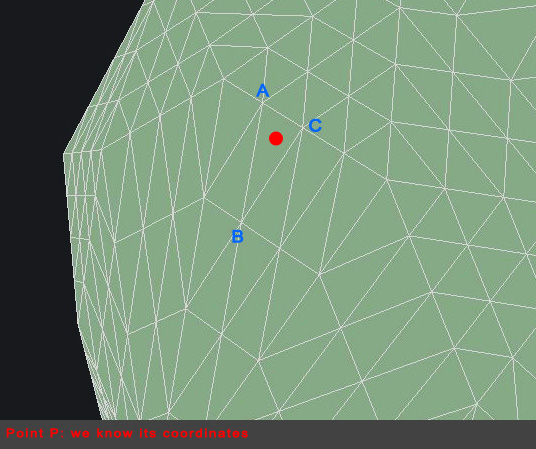# normal math

I have an irregular 3D mesh (meaning that is not a regular grid) and I have a glReadPixel routine that return the x,y,z coordinates of a point P clicked on the 3D mesh.
I’d like to calculate the normal for that point P, and the x,y,z rotation angles, so that I can display the normal.

First of all, I retrieve the 3 points closest to point P iterating all vertices and comparing non-square distance.
Once I have the closest points (ABC), I calculate the normal vector to the triangle like this:

normalVector = (C-A)^(B-A); // ^ is the cross product

I’m not sure this calculation is right because probably the order of vector subtractions matters.
Also, since I know the ABC normal values (the mesh it’s actually a .obj file with normals embedded), maybe it would be better to calculate point P normal interpolating the ABC normals?

And once I have calculated the correct normal, how would I calculate the proper xyz rotations out of it?

how would I calculate the proper xyz rotations out of it

Points do not have “proper xyz rotations”. What are you trying to accomplish with that?

i dont know if that equation is correct, but if i were you i’d just try it, and if you see the normal is pointing in the wrong direction, just change the order in your equation, i.e. make it (B-A)^(C-A). In any case you should also normalize the result, because if you can’t guarantee that C-A and B-A are of unit lenght, the normalVector wont be either. Also this sounds like a really slow algorithm to find the normals (iterating over all vertices and comparing distances to P).

Like that you will be able to calculate the normal at an arbitrary position in your mesh afaik, not only at vertices or face normals, but at any fragment.
It’s not a “better” solution, it simply depends on what you want to achieve. face normals or ‘fragment’ normals?

you mean something like euler angle orientation of the normal vector? I did a similar thing for calculating orientation of a camera given a vector describing the ‘direction’ of the camera (that is, (camera_position) - (look_at)).

``````
head = atan(dir.z / dir.x);
if(dir.x < 0)
pitch = atan(dir.z / dir.y);
if(dir.y < 0)
pitch += Pi;
``````

This should also work for your normal vectors (dir would be equal to the normal then). There should be a similar equation for calculating the roll parameter.

However that’s not something you’re going to need if you just want to display the normals.

I hope i haven’t written too many wrong things here, that’s just my understanding of the matterSorry for not being more precise. Here is a screenshot: we have a point P, whose coordinates are known (retrieved with glReadPixels).
I’d like to calculate the normal of the triangle where P is laying.
To do that, I need to find ABC, but as you can see it’s not a regular grid and I don’t understand how could I get ABC coordinates. Finding closest 3 points won’t help, because that wouldn’t mean that P is actually inside those.
Mmmhhhh kinda lost here…This statement changes the problem as you first stated it. I assume that you have the vertex coordinates of all the triangles being drawn. You also have the x,y,z coordinates of point P. All you have to do now is loop through the triangles making up the surface and test to see which one contains point P. Google ‘Point In Triangle’ to get more info. There are many different approaches. Once you identify the key triangle, it’s easy to calculate the normal. Sounds like you already know how to do this. Why is it that you want an Euler sequence for the normal? Is it to draw the normal?

How about rendering the normals (nx, ny, nz) as colors (R, G, B) using a fragment shader in an offscreen buffer and pick the value from here ?
Then for display you draw the segment from p to p+n or p + lambda * n to change the displayed length.

Max, I think the solution you suggested is the one I will follow. Thanks to everybody for your kind help!

Also this is a nice solution, thanks!